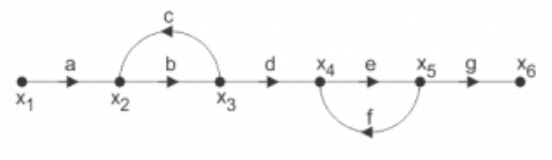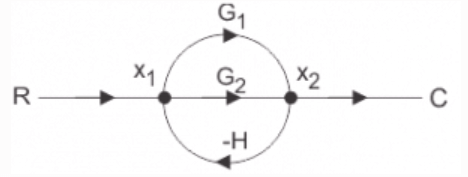# Signal Flow Graphs Objective Questions

Signal Flow Graphs Objective Questions

1. A signal flow graph is the graphical representation of the relationships between the variables of set linear algebraic equations.

a) True
b) False

Explanation: By definition signal flow graphs are the graphical representation of the relationships between the variables of set linear algebraic equations.

2. A node having only outgoing branches.

a) Input node
b) Output node
c) Incoming node
d) Outgoing node

Explanation: Nodes are the point by which the branches are outgoing or ingoing and this can be input or output node and input node is the node having only outgoing branches.

3. Use mason’s gain formula to find the transfer function of the given signal flow graph:a) abd/1-(ac)
b) abdeg/1-(bc+ef)+bcef
c) abd/1-(bc+ef)+bcef

Explanation: Using mason’s gain formula transfer function from signal flow graph can be calculated which relates the forward path gain to the various paths and loops.

4. Use mason’s gain formula to find the transfer function of the following signal flow graph:a) abcd+efg/1-cd-fg-cdfg
b) acdfg+bcefg/1-cd-fg-cdfg
c) abef+bcd/1-cd-fg-cdfg

Explanation: Using mason’s gain formula transfer function from signal flow graph can be calculated which relates the forward path gain to the various paths and loops.

5. Loop which do not possess any common node are said to be ___________ loops.

a) Forward gain
b) Touching loops
c) Non touching loops
d) Feedback gain

Explanation: Loop is the part of the network in which the branch starts from the node and comes back to the same node and non touching loop must not have any node in common.

6. Signal flow graphs:

a) They apply to linear systems
b) The equation obtained may or may not be in the form of cause or effect
c) Arrows are not important in the graph
d) They cannot be converted back to block diagram

Explanation: Signal flow graphs are used to find the transfer function of control system by converting the block diagrams into signal flow graphs or directly but cannot be used for nonlinear systems.

7. Signal flow graphs are reliable to find transfer function than block diagram reduction technique.

a) True
b) False

Explanation: As one set technique and formula is used here but in block diagram technique various methods are involved which increases complexity.

8. The relationship between an input and output variable of a signal flow graph is given by the net gain between the input and output node is known as the overall______________

a) Overall gain of the system
b) Stability
c) Bandwidth
d) Speed

Explanation: The relationship between input and output variable of a signal flow graph is the overall gain of the system.

9. Use mason’s gain formula to calculate the transfer function of given figure:a) G1/1+G2H
b) G1+G2/1+G1H
c) G2/1+G1H
d) None of the mentioned

Explanation: Use mason’s gain formula to solve the signal flow graph and by using mason’s gain formula transfer function from signal flow graph can be calculated which relates the forward path gain to the various paths and loops.

10. Use mason’s gain formula to find the transfer function of the given figure:a) G1+G2
b) G1+G1/1-G1H+G2H
c) G1+G2/1+G1H+G2H
d) G1-G2